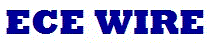Date: 14/12/2009

MATLAB; an essential tool for a serious engineer

MATLAB bolti kya' (do you speak MATLAB), this is what the advertisement put on the cover page of IEEE spectrum magazine by Mathworks reads in Hindi to promote its MATLAB tool. There is no question about its popularity; it is an engineer's favorite tool. True to its words, MATLAB is a key part of learning for today's engineers.

Engineering design is much about managing variables with some known pattern. The variable can be discrete or continuous. Figuring out visually how the 'n' number of related variable behaves over time and space requires lot of imagination inside the engineer's brain. Today's computers have more than sufficient computing power to offload such analytical works from engineer's brain. It's huge opportunity for software developers to tap into this market of design automation tools. Mathworks leads in the market of software tools for engineers and scientists through it's two major software packages MATLAB and SIMULINK.

MATLAB is an advanced software tool, comparable as current equivalent to previous generation's ubiquitous scientific calculator, which engineers could not work/study without it. MATLAB is the most used software by widebase of engineers.

In a candid discussion with this writer, Mathworks India's Managing Director Kishore Rao and Technical Manager Prashant Rao share the relevance of this tool for students and their efforts to make this tool even more popular in India.

In India Mathworks gets 30% of its revenues from Institutes and colleges, clearly indicating the role this tool is playing as part of engineering education. Both suggest Mathworks' products serve the needs of wide range of science and engineering areas such as core engineering, biotechnology, and also in financial services.

MATLAB does the computing tasks by using high level programming language with higher abstraction, where as SIMULINK is used for modeling and simulating the behavior of engineering systems. Both the tools houses huge library of application specific data for different application domains.

The application domains these tools can handle are broadly classified as Numerical Analysis, Linear Algebra, Control Design, Signal Processing, and Image Processing.

MATLAB is widely used by researchers and product innovators all around the world for designing solar powered cars to space-vehicles to theater design.

As per Mathworks, the advantages of their tools for technical education are, huge number of libraries, can be used for simple to complex problem solving and the theory can be quickly turned into practical experiments.

World's premier institutes including our IITs use Mathworks tools extensively in their research. Nearly every engineering college in India teaches MATLAB to their students.

Here is a nice comment from biology related scientific institute, Medical University of Carolina, "MATLAB enables young biologists to learn enough programming and math without being afraid of the code . They can write in MATLAB as if it were English"

In these days, for a job seeker, skill in using software tools matters a lot. The fluency in using tools such as MATLAB and SIMULINK is becoming a measuring yard for some of the employers, particularly in electronics and communications engineering.

Mathworks offers an online exchange called MATLAB Central for engineers to exchange and share information related to MATLAB. The MATLAB Central gets 662,000 visits per month. Also there are 100s of books on MATLAB and SIMULINK, some of them written by Indian authors. Mathworks also conducts classes both for faculty and students.

Kishore Rao says, "MATLAB is an intuitive and graphical tool to deal with the subsystem the engineers imagine."
"If you look at an educational environment, students are taught theory and concept as fundamentals. The tools like MATLAB allows them to visualize those learned concepts and theory on software and can look at the result in a better aspect. These tools allows engineers to simulate and test the concepts. Say, there is an equation and the data, engineers can run the data on the equation to see how the result are and see what happens if each parameters of the equation is changed.".

Prashanth Rao says, "typically, to do technical computing, engineers have to gather data from different files, from other software, hardware, and through data acquisition systems. MATLAB helps to analyze and explore the data, can model the data, and engineers can develop algorithms to work on this data and at the end, this can be deployed in any end-application."

"MATLAB lessens the programming barrier generally required in using scientific software tools so that the engineers who don't prefer to learn programming language such as mechanical and civil engineers find MATLAB more easy to use." adds Kishore Rao.

To learn more on Mathworks tools visit www.mathworks.com